# Chapter 6 Simulation and Resampling

Chapter Preview. Simulation is a computationally intensive method used to solve difficult problems. Instead of creating physical processes and experimenting with them in order to understand their operational characteristics, a simulation study is based on a computer representation - it considers various hypothetical conditions as inputs and summarizes the results. Through simulation, a vast number of hypothetical conditions can be quickly and inexpensively examined. Section 6.1 introduces simulation, a wonderful computational tool that is especially useful in complex, multivariate settings.

We can also use simulation to draw from an empirical distribution - this process is known as resampling. Resampling allows us to assess the uncertainty of estimates in complex models. Section 6.2 introduces resampling in the context of bootstrapping to determine the precision of estimators.

Subsequent sections introduce other topics in resampling. Section 6.3 on cross-validation shows how to use it for model selection and validation. Section 6.4 on importance sampling describes resampling in specific regions of interest, such as long-tailed actuarial applications. Section 6.5 on Monte Carlo Markov Chain (MCMC) introduces the simulation and resampling engine underpinning much of modern Bayesian analysis.

## 6.1 Simulation Fundamentals

In this section, you learn how to:

• Generate approximately independent realizations that are uniformly distributed
• Transform the uniformly distributed realizations to observations from a probability distribution of interest
• Calculate quantities of interest and determining the precision of the calculated quantities

### 6.1.1 Generating Independent Uniform Observations

The that we consider are generated by computers. A major strength of this approach is that they can be replicated, allowing us to check and improve our work. Naturally, this also means that they are not really random. Nonetheless, algorithms have been produced so that results appear to be random for all practical purposes. Specifically, they pass sophisticated tests of independence and can be designed so that they come from a single distribution - our assumption, identically and independently distributed.

To get a sense as to what these algorithms do, we consider a historically prominent method.

Linear Congruential Generator. To generate a sequence of random numbers, start with $B_0$, a starting value that is known as a seed. This value is updated using the recursive relationship $B_{n+1} = a B_n + c \text{ modulo }m, ~~ n=0, 1, 2, \ldots .$ This algorithm is called a . The case of $c=0$ is called a multiplicative congruential generator; it is particularly useful for really fast computations.

For illustrative values of $a$ and $m$, Microsoft’s Visual Basic uses $m=2^{24}$, $a=1,140,671,485$, and $c = 12,820,163$ (see https://en.wikipedia.org/wiki/Linear_congruential_generator). This is the engine underlying the random number generation in Microsoft’s Excel program.

The sequence used by the analyst is defined as $U_n=B_n/m.$ The analyst may interpret the sequence {$U_{i}$} to be (approximately) identically and independently uniformly distributed on the interval (0,1). To illustrate the algorithm, consider the following.

Example 6.1.1. Illustrative Sequence. Take $m=15$, $a=3$, $c=2$ and $B_0=1$. Then we have:

step $n$ $B_n$ $U_n$
0 $B_0=1$
1 $B_1 =\mod(3 \times 1 +2) = 5$ $U_1 = \frac{5}{15}$
2 $B_2 =\mod(3 \times 5 +2) = 2$ $U_2 = \frac{2}{15}$
3 $B_3 =\mod(3 \times 2 +2) = 8$ $U_3 = \frac{8}{15}$
4 $B_4 =\mod(3 \times 8 +2) = 11$ $U_4 = \frac{11}{15}$

Sometimes computer generated random results are known as to reflect the fact that they are machine generated and can be replicated. That is, despite the fact that {$U_{i}$} appears to be i.i.d, it can be reproduced by using the same seed number (and the same algorithm).

Example 6.1.2. Generating uniform random numbers in R. The following code shows how to generate three uniform (0,1) numbers in R using the runif command. The set.seed() function sets the initial seed. In many computer packages, the initial seed is set using the system clock unless specified otherwise.

##### Three Uniform Random Variates
set.seed(2017)
U <- runif(3)
knitr::kable(U, digits=5, align = "c", col.names = "Uniform")
Uniform
0.92424
0.53718
0.46920

The linear congruential generator is just one method of producing pseudo-random outcomes. It is easy to understand and is (still) widely used. The linear congruential generator does have limitations, including the fact that it is possible to detect long-run patterns over time in the sequences generated (recall that we can interpret independence to mean a total lack of functional patterns). Not surprisingly, advanced techniques have been developed that address some of this method’s drawbacks.

### 6.1.2 Inverse Transform Method

With the sequence of uniform random numbers, we next transform them to a distribution of interest, say $F$. A prominent technique is the , defined as

$X_i=F^{-1}\left( U_i \right) .$

Here, recall from Section 4.1.1 that we introduced the inverse of the distribution function, $F^{-1}$, and referred to it also as the . Specifically, it is defined to be

$F^{-1}(y) = \inf_x ~ \{ F(x) \ge y \} .$

Recall that $\inf$ stands for infimum or the . It is essentially the smallest value of x that satisfies the inequality $\{F(x) \ge y\}$. The result is that the sequence {$X_{i}$} is approximately iid with distribution function $F$.

The inverse transform result is available when the underlying random variable is continuous, discrete or a hybrid combination of the two. We now present a series of examples to illustrate its scope of applications.

Example 6.1.3. Generating exponential random numbers. Suppose that we would like to generate observations from an exponential distribution with scale parameter $\theta$ so that $F(x) = 1 - e^{-x/\theta}$. To compute the inverse transform, we can use the following steps: \begin{aligned} y = F(x) &\Leftrightarrow y = 1-e^{-x/\theta} \\ &\Leftrightarrow -\theta \ln(1-y) = x = F^{-1}(y) . \end{aligned}

Thus, if $U$ has a uniform (0,1) distribution, then $X = -\theta \ln(1-U)$ has an exponential distribution with parameter $\theta$.

The following R code shows how we can start with the same three uniform random numbers as in Example 6.1.2 and transform them to independent exponentially distributed random variables with a mean of 10. Alternatively, you can directly use the rexp function in R to generate random numbers from the exponential distribution. The algorithm built into this routine is different so even with the same starting seed number, individual realizations will differ.

set.seed(2017)
U <- runif(3)
X1 <- -10*log(1-U)
set.seed(2017)
X2 <- rexp(3, rate = 1/10)
##### Three Uniform Random Variates
Uniform Exponential 1 Exponential 2
0.92424 25.80219 3.25222
0.53718 7.70409 8.47652
0.46920 6.33362 5.40176

Example 6.1.4. Generating Pareto random numbers. Suppose that we would like to generate observations from a Pareto distribution with parameters $\alpha$ and $\theta$ so that $F(x) = 1 - \left(\frac{\theta}{x+\theta} \right)^{\alpha}$. To compute the inverse transform, we can use the following steps:

\begin{aligned} y = F(x) &\Leftrightarrow 1-y = \left(\frac{\theta}{x+\theta} \right)^{\alpha} \\ &\Leftrightarrow \left(1-y\right)^{-1/\alpha} = \frac{x+\theta}{\theta} = \frac{x}{\theta} +1 \\ &\Leftrightarrow \theta \left((1-y)^{-1/\alpha} - 1\right) = x = F^{-1}(y) .\end{aligned}

Thus, $X = \theta \left((1-U)^{-1/\alpha} - 1\right)$ has a Pareto distribution with parameters $\alpha$ and $\theta$.

Inverse Transform Justification. Why does the random variable $X = F^{-1}(U)$ have a distribution function $F$?

##### Show A Snippet of Theory

We now consider some discrete examples.

Example 6.1.5. Generating Bernoulli random numbers. Suppose that we wish to simulate random variables from a Bernoulli distribution with parameter $p=0.85$.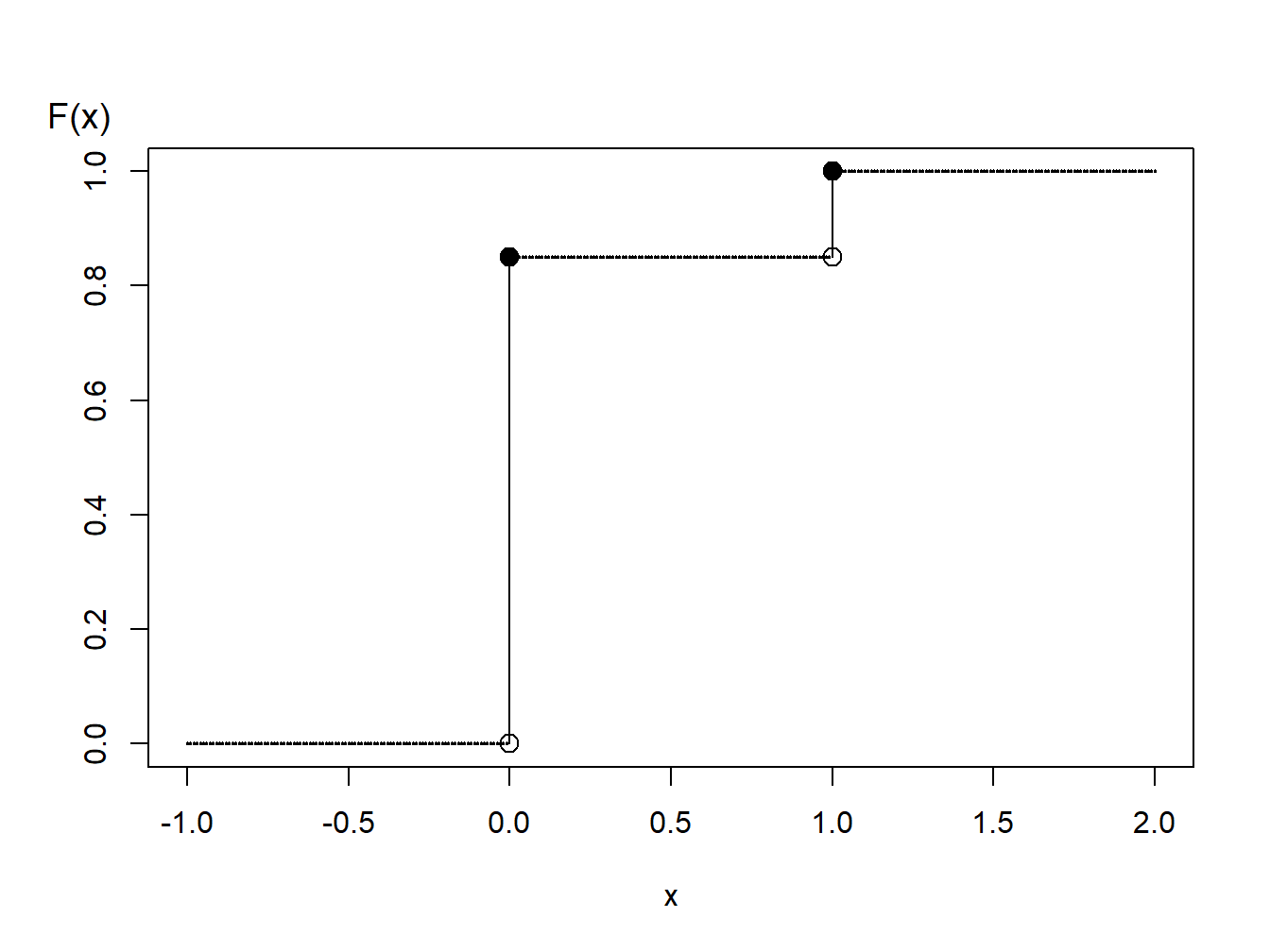Figure 6.1: Distribution Function of a Binary Random Variable

A graph of the cumulative distribution function in Figure 6.1 shows that the quantile function can be written as \begin{aligned} F^{-1}(y) = \left\{ \begin{array}{cc} 0 & 0<y \leq 0.85 \\ 1 & 0.85 < y \leq 1.0 . \end{array} \right. \end{aligned}

Thus, with the inverse transform we may define \begin{aligned} X = \left\{ \begin{array}{cc} 0 & 0<U \leq 0.85 \\ 1 & 0.85 < U \leq 1.0 \end{array} \right. \end{aligned} For illustration, we generate three random numbers to get

set.seed(2017)
U <- runif(3)
X <- 1*(U > 0.85)

#### Three Random Variates

Uniform Binary X
0.92424 1
0.53718 0
0.46920 0

Example 6.1.6. Generating random numbers from a discrete distribution. Consider the time of a machine failure in the first five years. The distribution of failure times is given as:

##### Discrete Distribution
$~~~~~~~~~~$ $~~~~~~~~~~$ $~~~~~~~~~~$ $~~~~~~~~~~$ $~~~~~~~~~~$
Time 1.0 2.0 3.0 4.0 5.0
Probability 0.1 0.2 0.1 0.4 0.2
Distribution Function $F(x)$ 0.1 0.3 0.4 0.8 1.0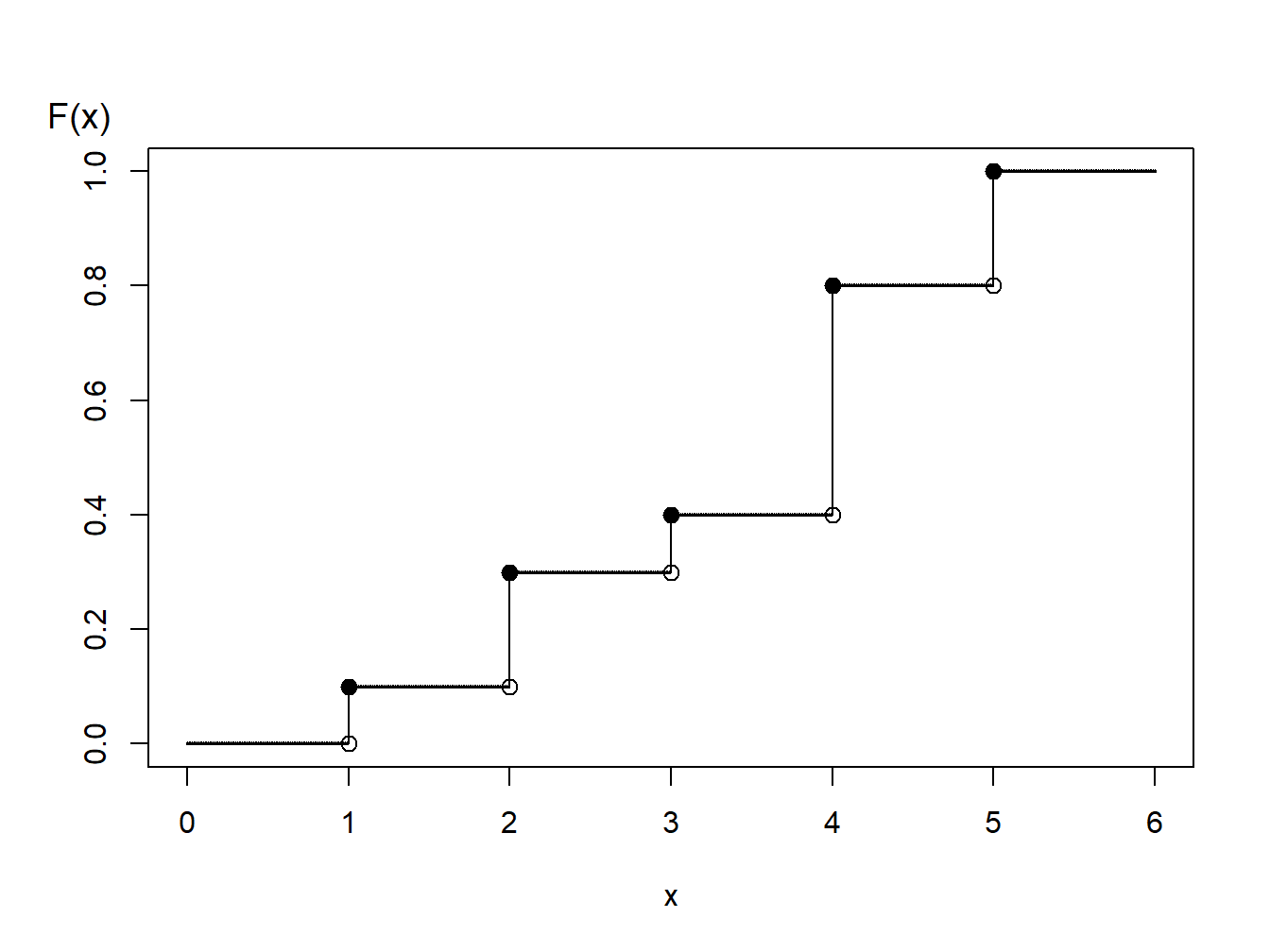Figure 6.2: Distribution Function of a Discrete Random Variable

Using the graph of the distribution function in Figure 6.2, with the inverse transform we may define

\small{ \begin{aligned} X = \left\{ \begin{array}{cc} 1 & 0<U \leq 0.1 \\ 2 & 0.1 < U \leq 0.3\\ 3 & 0.3 < U \leq 0.4\\ 4 & 0.4 < U \leq 0.8 \\ 5 & 0.8 < U \leq 1.0 . \end{array} \right. \end{aligned} }

For general discrete random variables there may not be an ordering of outcomes. For example, a person could own one of five types of life insurance products and we might use the following algorithm to generate random outcomes:

{\small \begin{aligned} X = \left\{ \begin{array}{cc} \textrm{whole life} & 0<U \leq 0.1 \\ \textrm{endowment} & 0.1 < U \leq 0.3\\ \textrm{term life} & 0.3 < U \leq 0.4\\ \textrm{universal life} & 0.4 < U \leq 0.8 \\ \textrm{variable life} & 0.8 < U \leq 1.0 . \end{array} \right. \end{aligned} }

Another analyst may use an alternative procedure such as:

{\small \begin{aligned} X = \left\{ \begin{array}{cc} \textrm{whole life} & 0.9<U<1.0 \\ \textrm{endowment} & 0.7 \leq U < 0.9\\ \textrm{term life} & 0.6 \leq U < 0.7\\ \textrm{universal life} & 0.2 \leq U < 0.6 \\ \textrm{variable life} & 0 \leq U < 0.2 . \end{array} \right. \end{aligned} }

Both algorithms produce (in the long-run) the same probabilities, e.g., $\Pr(\textrm{whole life})=0.1$, and so forth. So, neither is incorrect. You should be aware that “there is more than one way to skin a cat.” (What an old expression!) Similarly, you could use an alternative algorithm for ordered outcomes (such as failure times 1, 2, 3, 4, or 5, above).

Example 6.1.7. Generating random numbers from a hybrid distribution. Consider a random variable that is 0 with probability 70% and is exponentially distributed with parameter $\theta= 10,000$ with probability 30%. In an insurance application, this might correspond to a 70% chance of having no insurance claims and a 30% chance of a claim - if a claim occurs, then it is exponentially distributed. The distribution function, depicted in Figure 6.3, is given as

\begin{aligned} F(y) = \left\{ \begin{array}{cc} 0 & x<0 \\ 1 - 0.3 \exp(-x/10000) & x \ge 0 . \end{array} \right. \end{aligned}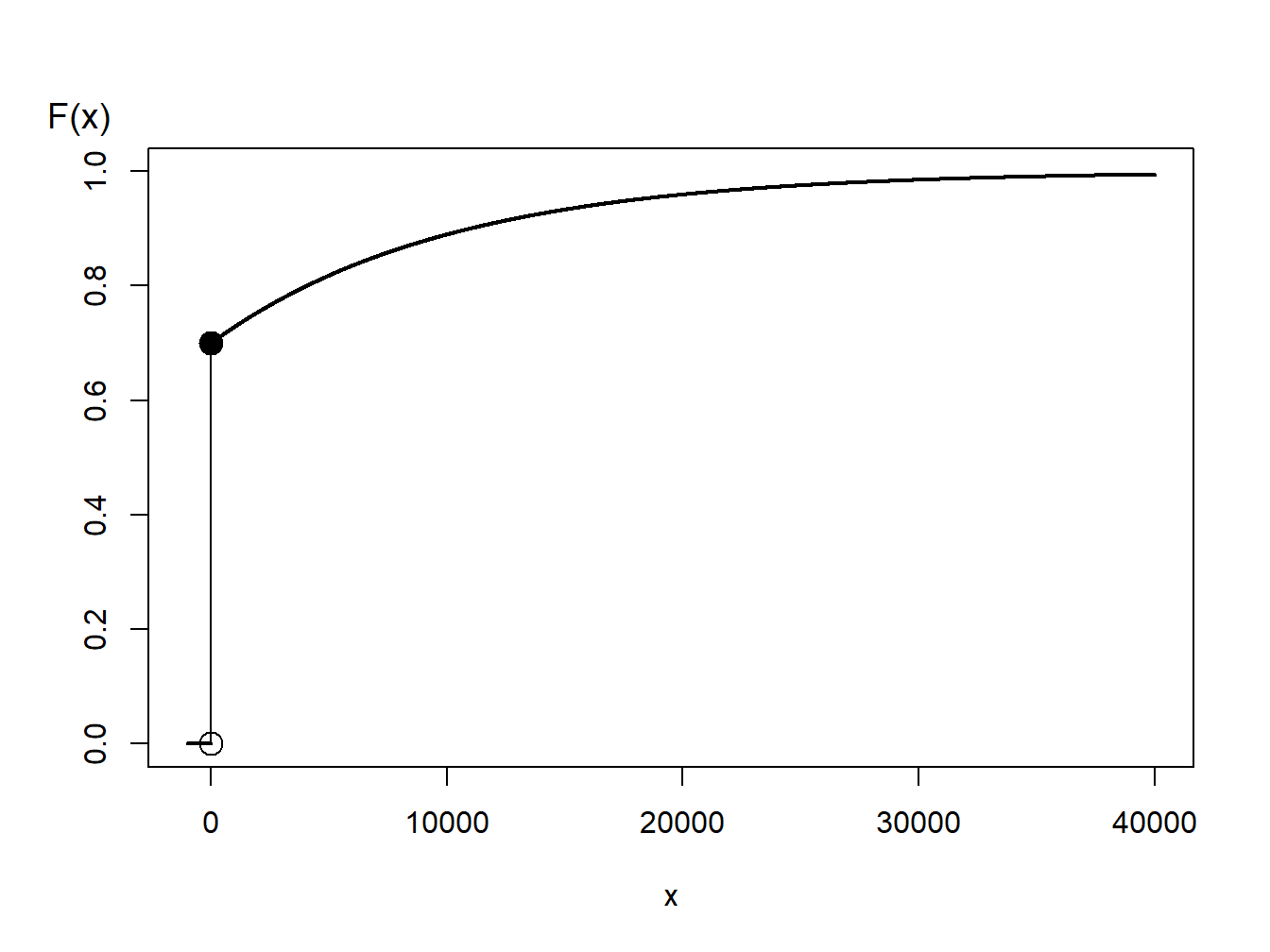Figure 6.3: Distribution Function of a Hybrid Random Variable

From Figure 6.3, we can see that the inverse transform for generating random variables with this distribution function is

\begin{aligned} X = F^{-1}(U) = \left\{ \begin{array}{cc} 0 & 0< U \leq 0.7 \\ -1000 \ln (\frac{1-U}{0.3}) & 0.7 < U < 1 . \end{array} \right. \end{aligned}

For discrete and hybrid random variables, the key is to draw a graph of the distribution function that allows you to visualize potential values of the inverse function.

### 6.1.3 Simulation Precision

From the prior subsections, we now know how to generate independent simulated realizations from a distribution of interest. With these realizations, we can construct an empirical distribution and approximate the underlying distribution as precisely as needed. As we introduce more actuarial applications in this book, you will see that simulation can be applied in a wide variety of contexts.

Many of these applications can be reduced to the problem of approximating $\mathrm{E~}h(X)$, where $h(\cdot)$ is some known function. Based on $R$ simulations (replications), we get $X_1,\ldots,X_R$. From this simulated sample, we calculate an average

$\overline{h}_R=\frac{1}{R}\sum_{i=1}^{R} h(X_i)$ that we use as our simulated approximate (estimate) of $\mathrm{E~}h(X)$. To estimate the precision of this approximation, we use the simulation variance

$s_{h,R}^2 = \frac{1}{R-1} \sum_{i=1}^{R}\left( h(X_i) -\overline{h}_R \right) ^2.$

From the independence, the standard error of the estimate is $s_{h,R}/\sqrt{R}$. This can be made as small as we like by increasing the number of replications $R$.

Example. 6.1.8. Portfolio management. In Section 3.4, we learned how to calculate the expected value of policies with deductibles. For an example of something that cannot be done with closed form expressions, we now consider two risks. This is a variation of a more complex example that will be covered as Example 10.3.6.

We consider two property risks of a telecommunications firm:

• $X_1$ - buildings, modeled using a gamma distribution with mean 200 and scale parameter 100.
• $X_2$ - motor vehicles, modeled using a gamma distribution with mean 400 and scale parameter 200.

Denote the total risk as $X = X_1 + X_2.$ For simplicity, you assume that these risks are independent.

To manage the risk, you seek some insurance protection. You wish to manage internally small building and motor vehicles amounts, up to $M$, say. Your retained risk is $Y_{retained}=$ $\min(X_1 + X_2,M)$. The insurer’s portion is $Y_{insurer} = X- Y_{retained}$.

To be specific, we use $M=$ 400 as well as $R=$ 1000000 simulations.

a. With the settings, we wish to determine the expected claim amount and the associated standard deviation of (i) that retained, (ii) that accepted by the insurer, and (iii) the total overall amount.

##### Show R Code to Define the Risks

Here is the code for the expected claim amounts.

##### Show R Code To Compute Amounts

The results of these calculations are:

round(outMat,digits=2)
                   Retained Insurer  Total
Mean                 365.17  235.01 600.18
Standard Deviation    69.51  280.86 316.36

b. For insured claims, the standard error of the simulation approximation is $s_{h,R}/\sqrt{1000000} =$ 280.86 $/\sqrt{1000000} =$ 0.281. For this example, simulation is quick and so a large value such as 1000000 is an easy choice. However, for more complex problems, the simulation size may be an issue.

##### Show R Code To Set up the Visualization

Figure 6.4 allows us to visualize the development of the approximation as the number of simulations increases.

matplot(1:R,sumP2[,1:20],type="l",col=rgb(1,0,0,.2), ylim=c(100, 400),
xlab=expression(paste("Number of Simulations (", italic('R'), ")")), ylab="Expected Insurer Claims")
abline(h=mean(Yinsurer),lty=2)
bonds <- cbind(1.96*sd(Yinsurer)*sqrt(1/(1:R)),-1.96*sd(Yinsurer)*sqrt(1/(1:R)))
matlines(1:R,bonds+mean(Yinsurer),col="red",lty=1)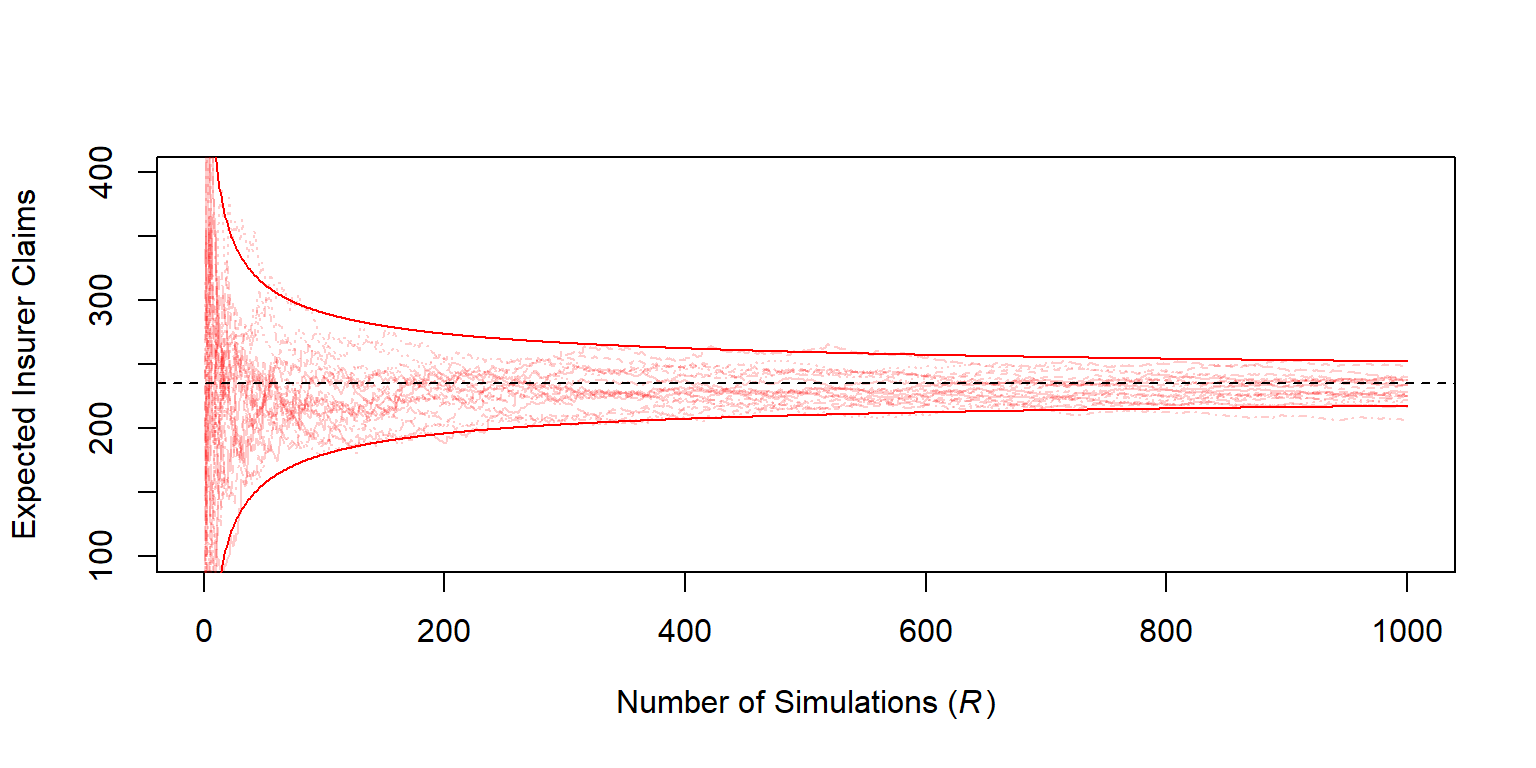Figure 6.4: Estimated Expected Insurer Claims versus Number of Simulations.

Determination of Number of Simulations

How many simulated values are recommended? 100? 1,000,000? We can use the to respond to this question.

As one criterion for your confidence in the result, suppose that you wish to be within 1% of the mean with 95% certainty. That is, you want $\Pr \left( |\overline{h}_R - \mathrm{E~}h(X)| \le 0.01 \mathrm{E~}h(X) \right) \le 0.95$. According to the central limit theorem, your estimate should be approximately normally distributed and so we want to have $R$ large enough to satisfy $0.01 \mathrm{E~}h(X)/\sqrt{\mathrm{Var~}h(X)/R}) \ge 1.96$. (Recall that 1.96 is the 97.5th percentile from the standard normal distribution.) Replacing $\mathrm{E~}h(X)$ and $\mathrm{Var~}h(X)$ with estimates, you continue your simulation until

$\frac{.01\overline{h}_R}{s_{h,R}/\sqrt{R}}\geq 1.96$ or equivalently

$\begin{equation} R \geq 38,416\frac{s_{h,R}^2}{\overline{h}_R^2}. \tag{6.1} \end{equation}$

This criterion is a direct application of the approximate normality. Note that $\overline{h}_R$ and $s_{h,R}$ are not known in advance, so you will have to come up with estimates, either by doing a small pilot study in advance or by interrupting your procedure intermittently to see if the criterion is satisfied.

Example. 6.1.8. Portfolio management - continued

For our example, the average insurance claim is 235.011 and the corresponding standard deviation is 280.862. Using equation (6.1), to be within 10% of the mean, we would only require at least 54.87 thousand simulations. However, to be within 1% we would want at least 5.49 million simulations.

Example. 6.1.9. Approximation choices. An important application of simulation is the approximation of $\mathrm{E~}h(X)$. In this example, we show that the choice of the $h(\cdot)$ function and the distribution of $X$ can play a role.

Consider the following question : what is $\Pr[X>2]$ when $X$ has a , with density $f(x) =\left(\pi(1+x^2)\right)^{-1}$, on the real line? The true value is

$\Pr\left[X>2\right] = \int_2^\infty \frac{dx}{\pi(1+x^2)} .$ One can use an R numerical integration function (which works usually well on improper integrals)

##### Show R Code for Parametric Bootstrap Samples

Figure 6.11 compares the bootstrap distributions for the coefficient of variation, one based on the nonparametric approach and the other based on a parametric approach, assuming a lognormal distribution.

plot(density(CV[,1]),col="red",main="",xlab="Coefficient of Variation", lty=1)
lines(density(CV[,2]),col="blue",lty=2)
abline(v=sd(sample_x)/mean(sample_x),lty=3)
legend("topright",c("nonparametric","parametric(LN)"),
col=c("red","blue"),lty=1:2,bty="n")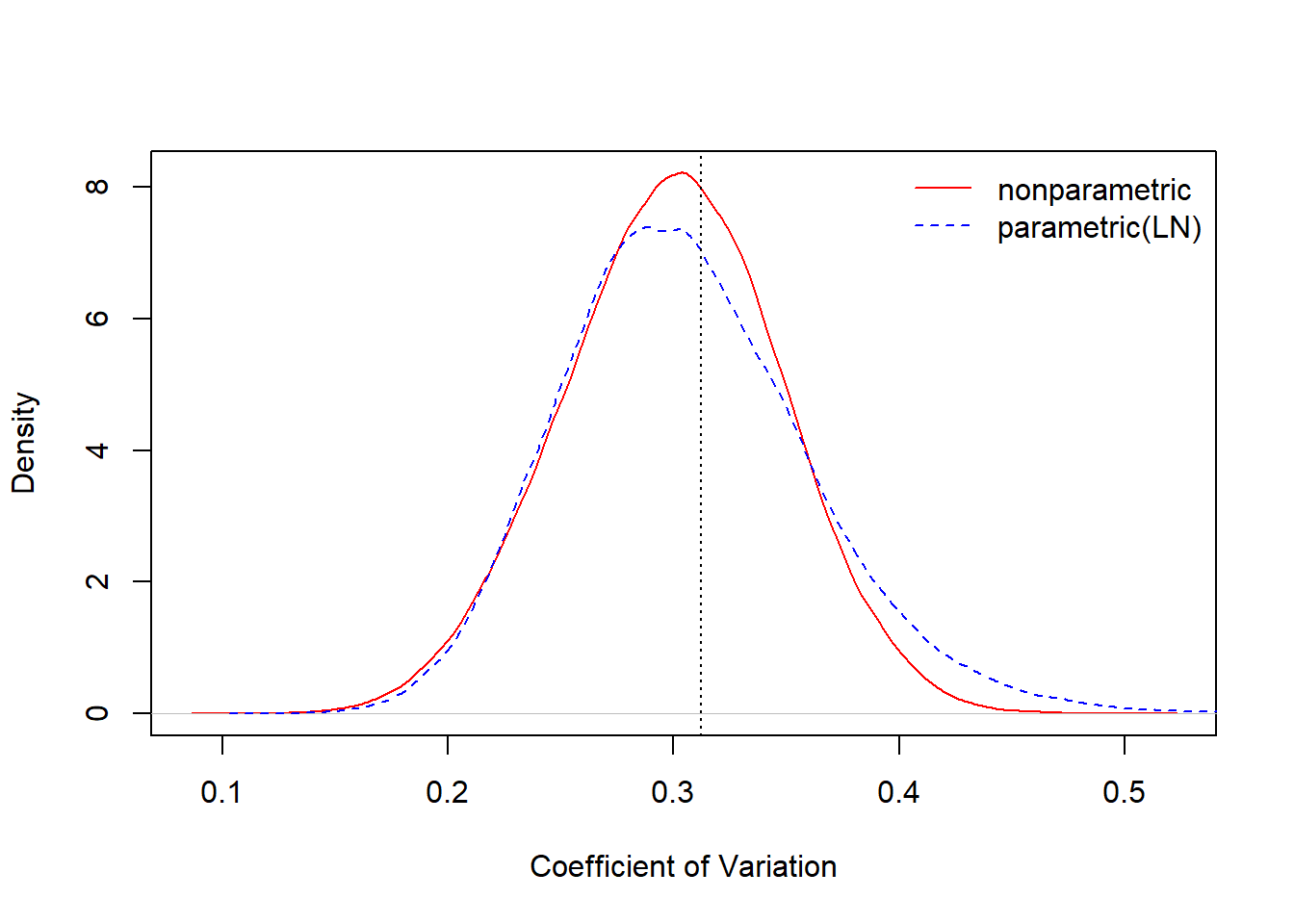Figure 6.11: Comparision of Nonparametric and Parametric Bootstrap Distributions for the Coefficient of Variation

Example 6.2.5. Bootstrapping Censored Observations. The parametric bootstrap draws simulated realizations from a parametric estimate of the distribution function. In the same way, we can draw simulated realizations from estimates of a distribution function. As one example, we might draw from smoothed estimates of a distribution function introduced in Section 4.1.1.4. Another special case, considered here, is to draw an estimate from the Kaplan-Meier estimator introduced in Section 4.3.2.2. In this way, we can handle observations that are censored.

Specifically, return to the bodily injury data in Examples 6.2.1 and 6.2.3 but now we include the 17 claims that were censored by policy limits. In Example 4.3.6, we used this full dataset to estimate the Kaplan-Meier estimator of the survival function introduced in Section 4.3.2.2. Table 6.6 present bootstrap estimates of the quantiles from the Kaplan-Meier survival function estimator. These include the bootstrap precision estimates, bias and standard deviation, as well as the basic 95% confidence interval.

##### Table 6.6. Bootstrap Kaplan-Meier Estimates of Quantiles at Selected Fractions
Fraction KM NP Estimate Bootstrap Bias Bootstrap SD Lower Basic 95% CI Upper Basic 95% CI
0.50 6500 13.58 181.23 6093 6923
0.80 9500 173.61 423.41 8445 9949
0.90 12756 20.17 675.86 10812 14012
0.95 18500 Inf NaN 12500 22300
0.98 25000 Inf NaN -Inf 27308

Results in Table 6.6 are consistent with the results for the uncensored subsample in Table 6.4. In Table 6.6, we note the difficulty in estimating quantiles at large fractions due to the censoring. However, for moderate size fractions (0.50, 0.80, and 0.90), the Kaplan-Meier nonparametric (KM NP) estimates of the quantile are consistent with those Table 6.4. The bootstrap standard deviation is smaller at the 0.50 (corresponding to the median) but larger at the 0.80 and 0.90 levels. The censored data analysis summarized in Table 6.6 uses more data than the uncensored subsample analysis in Table 6.4 but also has difficulty extracting information for large quantiles.

## 6.3 Cross-Validation

In this section, you learn how to:

• Compare and contrast cross-validation to simulation techniques and bootstrap methods.
• Use cross-validation techniques for model selection
• Explain the jackknife method as a special case of cross-validation and calculate jackknife estimates of bias and standard errors

Cross-validation, briefly introduced in Section 4.2.4, is a technique based on simulated outcomes and so let’s think about its purposes in contrast to other simulation techniques already introduced in this chapter.

• Simulation, or Monte-Carlo, introduced in Section 6.1, allow us to compute expected values and other summaries of statistical distributions, such as $p$-values, readily.
• Bootstrap, and other resampling methods introduced in Section 6.2, provide estimators of the precision, or variability, of statistics.
• Cross-validation is important when assessing how accurately a predictive model will perform in practice.

Overlap exists but nonetheless it is helpful to think about the broad goals as associated with each statistical method.

To discuss cross-validation, let us recall from Section 4.2 some of the key ideas of model validation. When assessing, or validating, a model, we look to performance measured on new data, or at least not those that were used to fit the model. A classical approach, described in Section 4.2.3, is to split the sample in two: a subpart (the training dataset) is used to fit the model and another one (the testing dataset) is used to validate. However, a limitation of this approach is that results depend on the split; even though the overall sample is fixed, the split between training and test subsamples varies randomly. A different training sample means that model estimated parameters will differ. Different model parameters and a different test sample means that validation statistics will differ. Two analysts may use the same data and same models yet reach different conclusions about the viability of a model (based on different random splits), a frustrating situation.

### 6.3.1 k-Fold Cross-Validation

To mitigate this difficulty, it is common to use a cross-validation approach as introduced in Section 4.2.4. The key idea is to emulate the basic test/training approach to model validation by repeating it many times through averaging over different splits of the data. A key advantage is that the validation statistic is not tied to a specific parametric (or nonparametric) model - one can use a nonparametric statistic or a statistic that has economic interpretations - and so this can be used to compare models that are not nested (unlike likelihood ratio procedures).

Example 6.3.1. Wisconsin Property Fund. For the 2010 property fund data introduced in Section 1.3, we fit gamma and Pareto distributions to the 1,377 claims data. For details of the related goodness of fit, see Appendix Section 15.4.4. We now consider the Kolmogorov-Smirnov statistic introduced in Section 4.1.2.2. When the entire dataset was fit, the Kolmogorov-Smirnov goodness of fit statistic for the gamma distribution turns out to be 0.0478 and for the Pareto distribution is 0.2639. The lower value for the Pareto distribution indicates that this distribution is a better fit than the gamma.

To see how works, we randomly split the data into $k=8$ groups, or folds, each having about $1377/8 \approx 172$ observations. Then, we fit gamma and Pareto models to a data set with the first seven folds (about $172*7 = 1204$ observations), determine estimated parameters, and then used these fitted models with the held-out data to determine the Kolmogorov-Smirnov statistic.

##### Show R Code for Kolmogorov-Smirnov Cross-Validation

The results appear in Figure 6.12 where horizontal axis is Fold=1. This process was repeated for the other seven folds. The results summarized in Figure 6.12 show that the Pareto consistently provides a more reliable predictive distribution than the gamma.

# Plot the statistics
matplot(1:k,t(cvalvec),type="b", col=c(1,3), lty=1:2,
ylim=c(0,0.4), pch = 0, xlab="Fold", ylab="KS Statistic")
legend("left", c("Pareto", "Gamma"), col=c(1,3),lty=1:2, bty="n")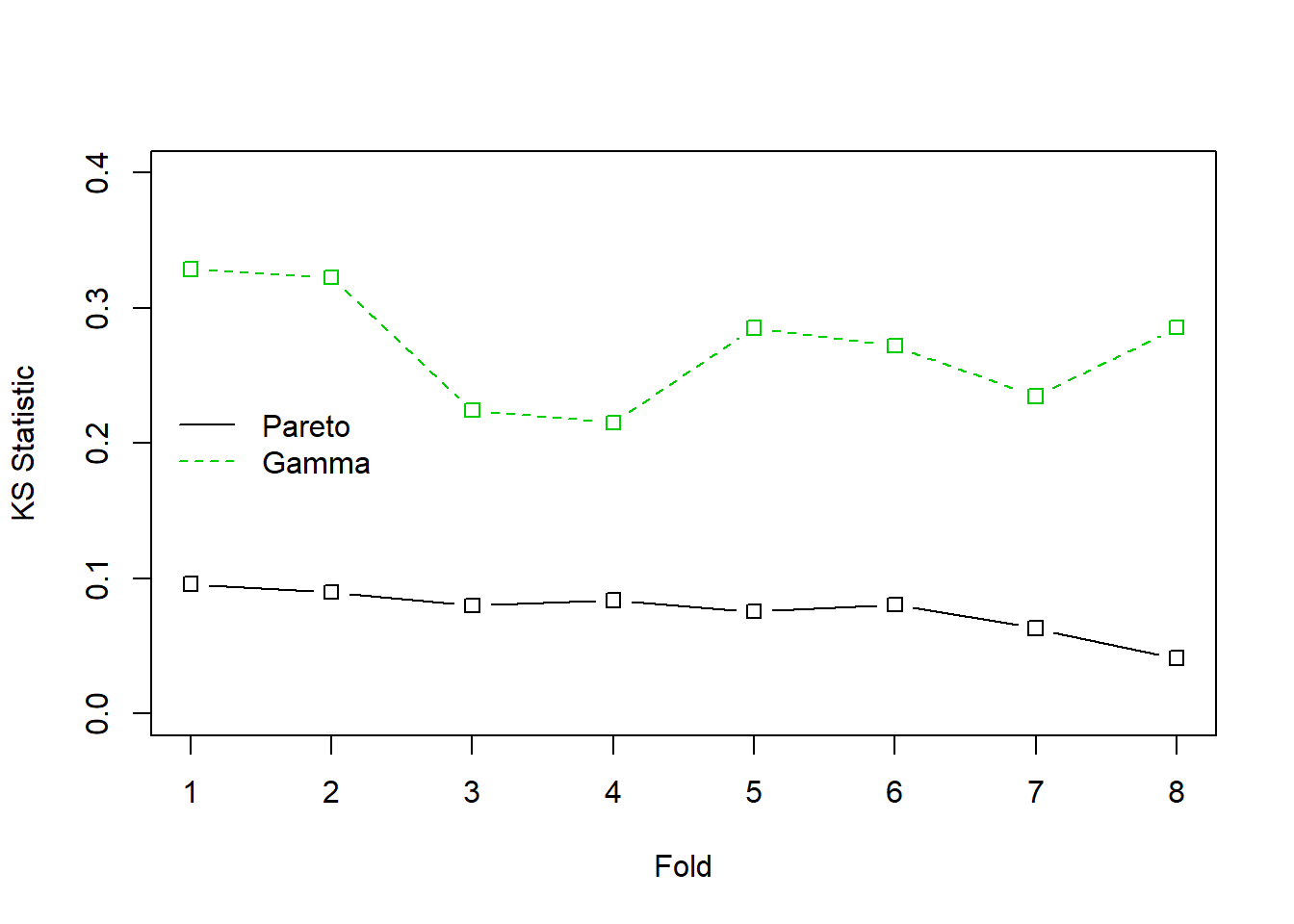Figure 6.12: Cross Validated Kolmogorov-Smirnov (KS) Statistics for the Property Fund Claims Data. The solid black line is for the Pareto distribution, the green dashed line is for the gamma distribution. The KS statistic measures the largest deviation between the fitted distribution and the empirical distribution for each of 8 groups, or folds, of randomly selected data.

### 6.3.2 Leave-One-Out Cross-Validation

A special case where $k=n$ is known as . This case is historically prominent and is closely related to , a precursor of the bootstrap technique.

Even though we present it as a special case of cross-validation, it is helpful to given an explicit definition. Consider a generic statistic $\widehat{\theta}=t(\boldsymbol{x})$ that is an estimator for a parameter of interest $\theta$. The idea of the jackknife is to compute $n$ values $\widehat{\theta}_{-i}=t(\boldsymbol{x}_{-i})$, where $\boldsymbol{x}_{-i}$ is the subsample of $\boldsymbol{x}$ with the $i$-th value removed. The average of these values is denoted as $\overline{\widehat{\theta}}_{(\cdot)}=\frac{1}{n}\sum_{i=1}^n \widehat{\theta}_{-i} .$ These values can be used to create estimates of the bias of the statistic $\widehat{\theta}$

$\begin{equation} Bias_{jack} = (n-1) \left(\overline{\widehat{\theta}}_{(\cdot)} - \widehat{\theta}\right) \tag{6.3} \end{equation}$

as well as a standard deviation estimate

$\begin{equation} s_{jack} =\sqrt{\frac{n-1}{n}\sum_{i=1}^n \left(\widehat{\theta}_{-i} -\overline{\widehat{\theta}}_{(\cdot)}\right)^2} ~. \tag{6.4} \end{equation}$

Example 6.3.2. Coefficient of Variation. To illustrate, consider a small fictitious sample $\boldsymbol{x}=\{x_1,\ldots,x_n\}$ with realizations

sample_x <- c(2.46,2.80,3.28,3.86,2.85,3.67,3.37,3.40,
5.22,2.55,2.79,4.50,3.37,2.88,1.44,2.56,2.00,2.07,2.19,1.77)

Suppose that we are interested in the $\theta = CV = \sqrt{\mathrm{Var~}X}/\mathrm{E~}X$.

With this dataset, the estimator of the coefficient of variation turns out to be 0.31196. But how reliable is it? To answer this question, we can compute the jackknife estimates of bias and its standard deviation. The following code shows that the jackknife estimator of the bias is $Bias_{jack} =$ -0.00627 and the jackknife standard deviation is $s_{jack} =$ 0.01293.

CVar <- function(x) sqrt(var(x))/mean(x)
JackCVar <- function(i) sqrt(var(sample_x[-i]))/mean(sample_x[-i])
JackTheta <- Vectorize(JackCVar)(1:length(sample_x))
BiasJack <- (length(sample_x)-1)*(mean(JackTheta) - CVar(sample_x))
sd(JackTheta)

Example 6.3.3. Bodily Injury Claims and Loss Elimination Ratios. In Example 6.2.1, we showed how to compute bootstrap estimates of the bias and standard deviation for the loss elimination ratio using the Example 4.1.11 bodily injury claims data. We follow up now by providing comparable quantities using jackknife statistics.

Table 6.7 summarizes the results of the jackknife estimation. It shows that jackknife estimates of the bias and standard deviation of the loss elimination ratio $\mathrm{E}~\min(X,d)/\mathrm{E}~X$ are largely consistent with the bootstrap methodology. Moreover, one can use the standard deviations to construct normal based confidence intervals, centered around a bias-corrected estimator. For example, at $d=14000$, we saw in Example 4.1.11 that the nonparametric estimate of LER is 0.97678. This has an estimated bias of 0.00010, resulting in the (jackknife) bias-corrected estimator 0.97688. The 95% confidence intervals are produced by creating an interval of twice the length of 1.96 jackknife standard deviations, centered about the bias-corrected estimator (1.96 is the approximate 97.5th quantile of the standard normal distribution).

##### Table 6.7. Jackknife Estimates of LER at Selected Deductibles
d NP Estimate Bootstrap Bias Bootstrap SD Jackknife Bias Jackknife SD Lower Jackknife 95% CI Upper Jackknife 95% CI
4000 0.54113 -0.00012 0.01228 0.00031 0.00061 0.53993 0.54233
5000 0.64960 -0.00038 0.01400 0.00033 0.00068 0.64825 0.65094
10500 0.93563 0.00018 0.01065 0.00019 0.00053 0.93460 0.93667
11500 0.95281 -0.00016 0.00918 0.00016 0.00047 0.95189 0.95373
14000 0.97678 0.00018 0.00701 0.00010 0.00034 0.97612 0.97745
18500 0.99382 0.00006 0.00342 0.00003 0.00017 0.99350 0.99415

Discussion. One of the many interesting things about the leave-one-out special case is the ability to replicate estimates exactly. That is, when the size of the fold is only one, then there is no additional uncertainty induced by the cross-validation. This means that analysts can exactly replicate work of one another, an important consideration.

Jackknife statistics were developed to understand precision of estimators, producing estimators of bias and standard deviation in equations (6.3) and (6.4). This crosses into goals that we have associated with bootstrap techniques, not cross-validation methods. This demonstrates how statistical techniques can be used to achieve different goals.

### 6.3.3 Cross-Validation and Bootstrap

The bootstrap is useful in providing estimators of the precision, or variability, of statistics. It can also be useful for model validation. The bootstrap approach to model validation is similar to the leave-one-out and k-fold validation procedures:

• Create a bootstrap sample by re-sampling (with replacement) $n$ indices in $\{1,\cdots,n\}$. That will be our training sample. Estimate the model under consideration based on this sample.
• The test, or validation sample, consists of those observations not selected for training. Evaluate the fitted model (based on the training data) using the test data.

Repeat this process many (say $B$) times. Take an average over the results and choose the model based on the average evaluation statistic.

Example 6.3.4. Wisconsin Property Fund. Return to Example 6.3.1 where we investigate the fit of the gamma and Pareto distributions on the property fund data. We again compare the predictive performance using the Kolmogorov-Smirnov statistic but this time using the bootstrap procedure to split the data between training and testing samples. The following provides illustrative code.

##### Show R Code for Bootstrapping Validation Procedures

We did the sampling using $B=$ 100 replications. The average KS statistic for the Pareto distribution was 0.058 compared to the average for the gamma distribution, 0.261. This is consistent with earlier results and provides another piece of evidence that the Pareto is a better model for these data than the gamma.

## 6.4 Importance Sampling

Section 6.1 introduced Monte Carlo techniques using the inversion technique : to generate a random variable $X$ with distribution $F$, apply $F^{-1}$ to calls of a random generator (uniform on the unit interval). What if we what to draw according to $X$, conditional on $X\in[a,b]$ ?

• if $x\in[a,b]$ : keep it (“accept”)
• if $x\notin[a,b]$ : draw another one (“reject”)

Observe that from $n$ values initially generated, we keep here only $[F(b)-F(a)]\cdot n$ draws, on average.

Example 6.4.1. Draws from a Normal Distribution. Suppose that we draw from a normal distribution with mean 2.5 and variance 1, $N(2.5,1)$, but are only interested in draws greater that $a \ge 2$ and less than $b \le 4$. That is, we can only use $F(4)-F(2) = \Phi(4-2.5)-\Phi(2-2.5)$ = 0.9332 - 0.3085 = 0.6247 proportion of the draws. Figure 6.13 demonstrates that some draws lie with the interval $(2,4)$ and some are outside.

##### Show R Code for Accept-Reject Mechanism
for (i in 1:numAnimation) {pic_ani()}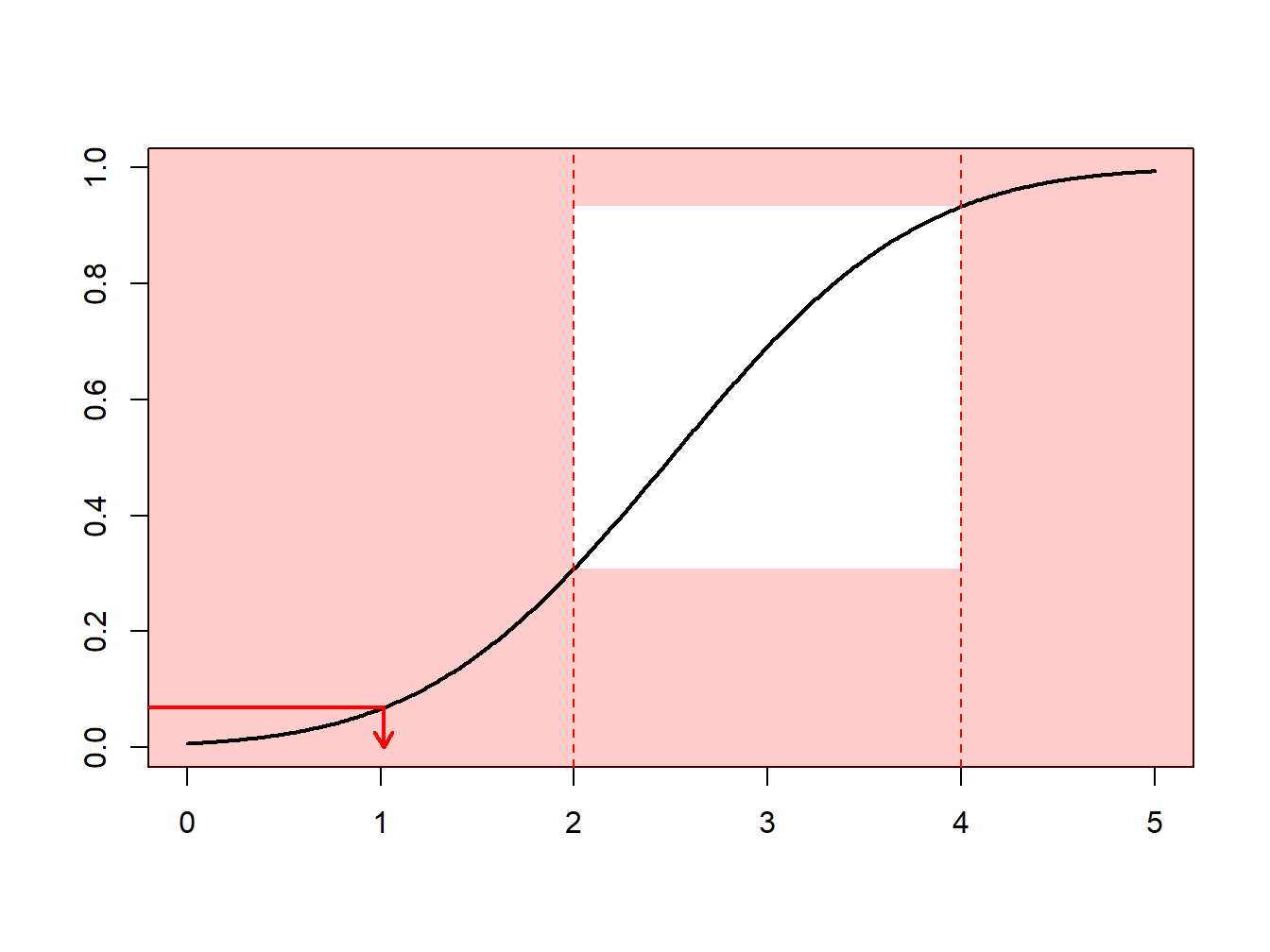Figure 6.13: Animated Demonstration of Draws In and Outside of (2,4)

Instead, one can draw according to the conditional distribution $F^{\star}$ defined as

$F^{\star}(x) = \Pr(X \le x | a < X \le b) =\frac{F(x)-F(a)}{F(b)-F(a)}, \ \ \ \text{for } a < x \le b .$

Using the inverse transform method in Section 6.1.2, we have that the draw

$X^\star=F^{\star-1}\left( U \right) = F^{-1}\left(F(a)+U\cdot[F(b)-F(a)]\right)$

has distribution $F^{\star}$. Expressed another way, define $\tilde{U} = (1-U)\cdot F(a)+U\cdot F(b)$ and then use $F^{-1}(\tilde{U})$. With this approach, each draw counts.

This can be related to the : we draw more frequently in regions where we expect to have quantities that have some interest. This transform can be considered as a “a change of measure.”

##### Show R Code for Importance Sampling by the Inverse Transform Method
for (i in 1:numAnimation) {pic_ani()}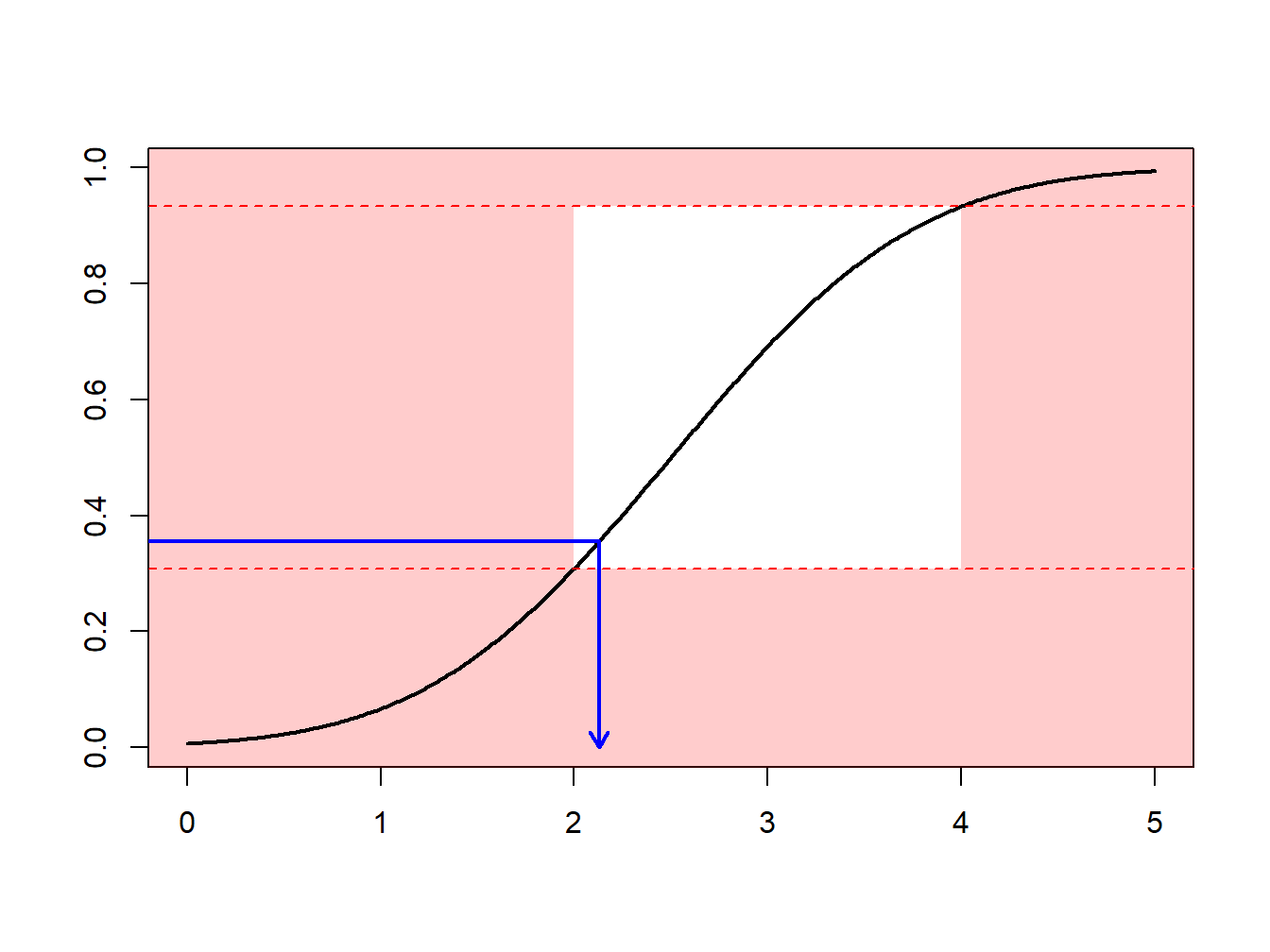In Example 6.4.1., the inverse of the normal distribution is readily available (in R, the function is qnorm). However, for other applications, this is not the case. Then, one simply uses numerical methods to determine $X^\star$ as the solution of the equation $F(X^\star) =\tilde{U}$ where $\tilde{U}=(1-U)\cdot F(a)+U\cdot F(b)$. See the following illustrative code.

##### Show R Code for Importance Sampling via Numerical Solutions
for (i in 1:numAnimation) {pic_ani()}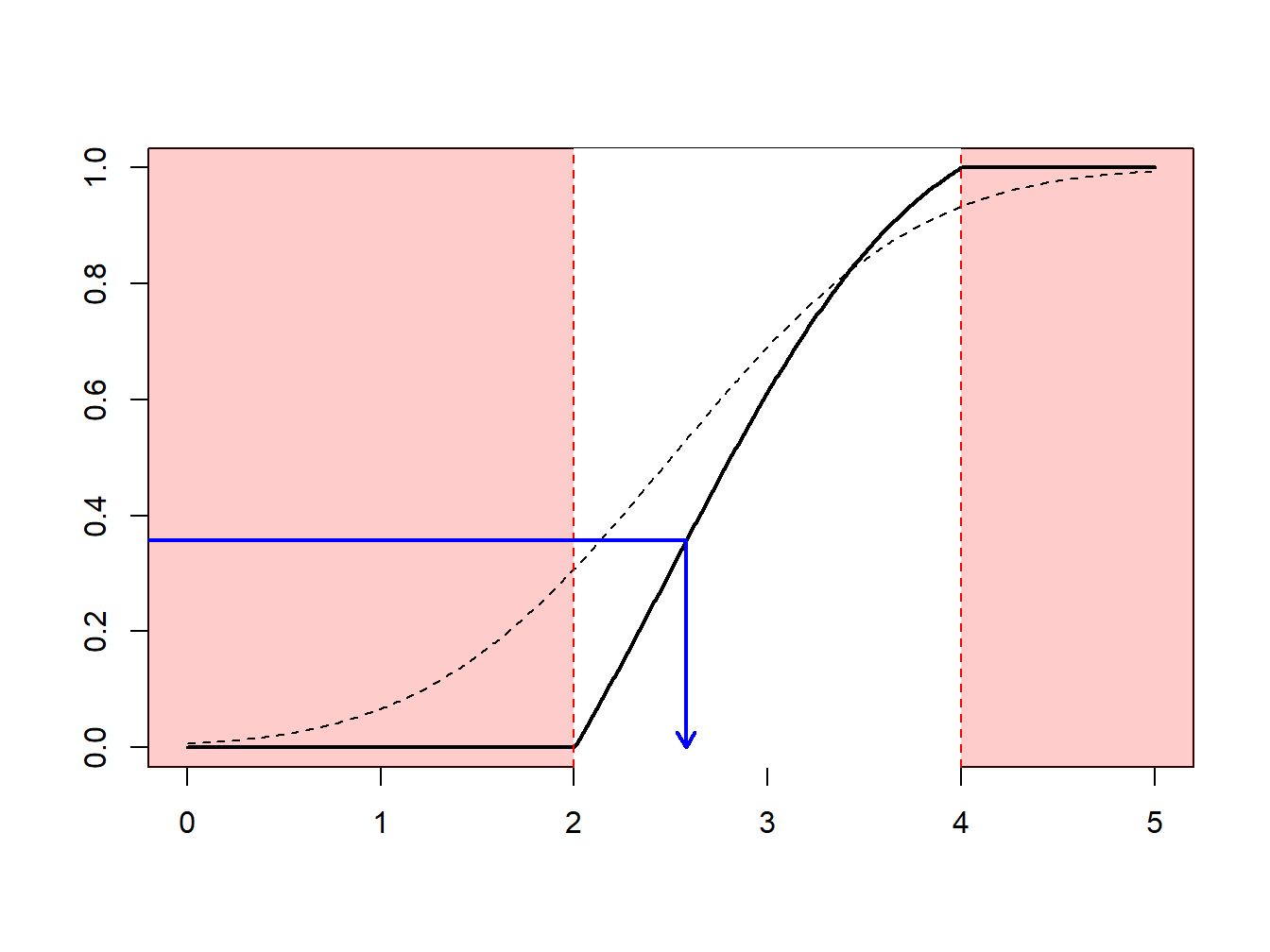## 6.5 Monte Carlo Markov Chain (MCMC)

This section is being written and is not yet complete nor edited. It is here to give you a flavor of what will be in the final version.

The idea of Monte Carlo techniques rely on the law of large number (that insures the convengence of the average towards the integral) and the central limit theorem (that is used to quantify uncertainty in the computations). Recall that if $(X_i)$ is an i.id. sequence of random variables with distribution $F$, then $\frac{1}{\sqrt{n}}\left(\sum_{i=1}^n h(X_i)-\int h(x)dF(x)\right)\overset{\mathcal{L}}{\rightarrow }\mathcal{N}(0,\sigma^2),\text{ as }n\rightarrow\infty.$ for some variance $\sigma^2>0$. But actually, the can be used to weaker the previous result, since it is not necessary to have independence of the variables. More precisely, if $(X_i)$ is a with $\mu$, under some additional technical assumptions, we can obtain that $\frac{1}{\sqrt{n}}\left(\sum_{i=1}^n h(X_i)-\int h(x)d\mu(x)\right)\overset{\mathcal{L}}{\rightarrow }\mathcal{N}(0,\sigma_\star^2),\text{ as }n\rightarrow\infty.$ for some variance $\sigma_\star^2>0$.

Hence, from this property, we can see that it is possible not necessarily to generate independent values from $F$, but to generate a markov process with invariante measure $F$, and to consider means over the process (not necessarily independent).

Consider the case of a constraint Gaussian vector : we want to generate random pairs from a random vector $\boldsymbol{X}$, but we are interested only on the case where the sum of the is large enough, which can be written $\boldsymbol{X}^T\boldsymbol{1}> m$ for some real valued $m$. Of course, it is possible to use the accept-reject algorithm, but we have seen that it might be quite inefficient. One can use and to generate a Markov process with such an invariant measure.

### 6.5.1 Hastings Metropolis

The algorithm is rather simple to generate from $f$ : we start with a feasible value $x_1$. Then, at step $t$, we need to specify a transition kernel : given $x_t$, we need a conditional distribution for $X_{t+1}$ given $x_t$. The algorithm will work well if that conditional distribution can easily be simulated. Let $\pi(\cdot|x_t)$ denote that probability.

Draw a potential value $x_{t+1}^\star$, and $u$, from a uniform distribution. Compute $R= \frac{f(x_{t+1}^\star)}{f(x_t)}$ and

• if $u < r$, then set $x_{t+1}=x_t^\star$
• if $u\leq r$, then set $x_{t+1}=x_t$

Here $r$ is called the acceptance-ratio: we accept the new value with probability $r$ (or actually the smallest between $1$ and $r$ since $r$ can exceed $1$).

For instance, assume that $f(\cdot|x_t)$ is uniform on $[x_t-\varepsilon,x_t+\varepsilon]$ for some $\varepsilon>0$, and where $f$ (our target distribution) is the $\mathcal{N}(0,1)$. We will never draw from $f$, but we will use it to compute our acceptance ratio at each step.

##### Show R Code

In the code above, vec contains values of $\boldsymbol{x}=(x_1,x_2,\cdots)$, innov is the innovation.

##### Show R Code
for (k in 2:23) {pic_ani(k)}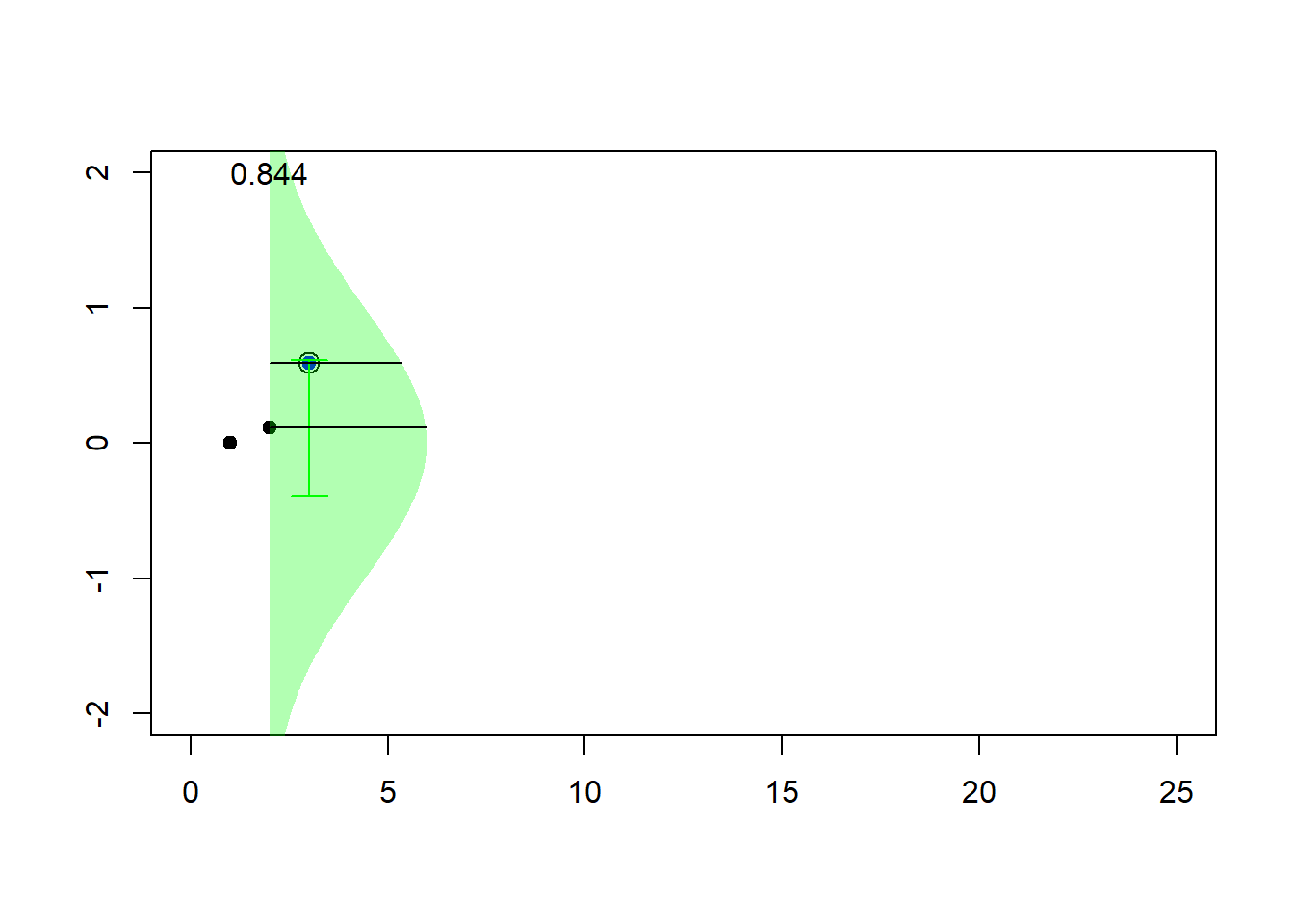Now, if we use more simulations, we get

vec <- metrop1(10000)
simx <- vec[1000:10000,1]
par(mfrow=c(1,4))
plot(simx,type="l")
hist(simx,probability = TRUE,col="light blue",border="white")
lines(u,dnorm(u),col="red")
qqnorm(simx)
acf(simx,lag=100,lwd=2,col="light blue")### 6.5.2 Gibbs sampler

Consider some vector $\boldsymbol{X}=(X_1,\cdots,X_d)$ with ind'ependent components, $X_i\sim\mathcal{E}(\lambda_i)$. We sample to sample from $\boldsymbol{X}$ given $\boldsymbol{X}^T\boldsymbol{1}>s$ for some threshold $s>0$.

• start with some starting point $\boldsymbol{x}_0$ such that
• pick up (randomly) $i\in\{1,\cdots,d\}$
• $X_i$ given $X_i > s-\boldsymbol{x}_{(-i)}^T\boldsymbol{1}$ has an Exponential distribution $\mathcal{E}(\lambda_i)$
• draw $Y\sim \mathcal{E}(\lambda_i)$ and set $x_i=y +(s-\boldsymbol{x}_{(-i)}^T\boldsymbol{1})_+$ until $\boldsymbol{x}_{(-i)}^T\boldsymbol{1}+x_i>s$
##### Show R Code
plot(sim,xlim=c(1,11),ylim=c(0,4.3))
polygon(c(-1,-1,6),c(-1,6,-1),col="red",density=15,border=NA)
abline(5,-1,col="red")The construction of the sequence ( algorithms are iterative) can be visualized below

##### Show R Code
for (i in 2:100) {pic_ani(i)}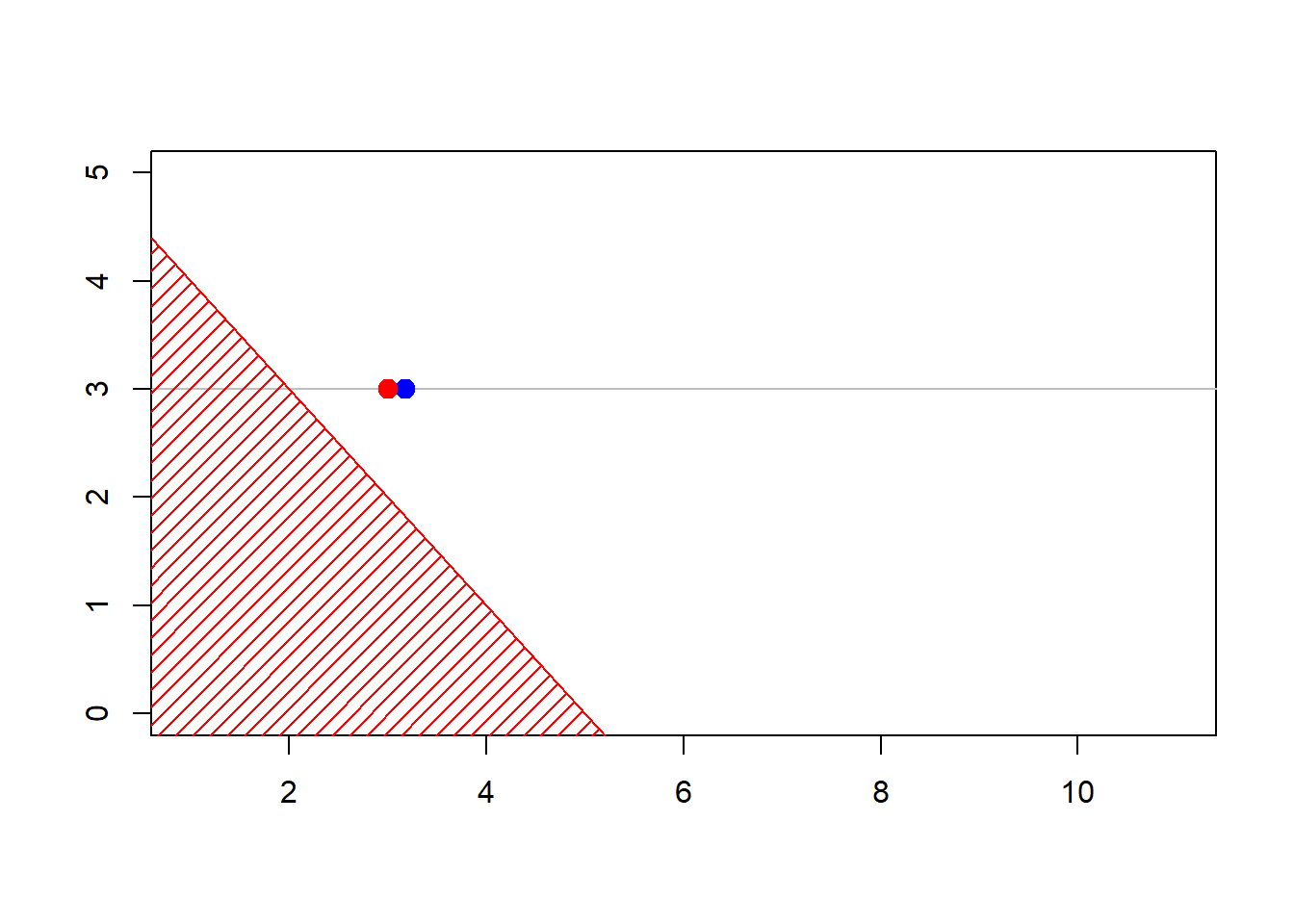## 6.6 Further Resources and Contributors

#### Contributors

• Arthur Charpentier, Université du Quebec á Montreal, and Edward W. (Jed) Frees, University of Wisconsin-Madison, are the principal authors of the initial version of this chapter. Email: jfrees@bus.wisc.edu and/or arthur.charpentier@gmail.com for chapter comments and suggested improvements.
• Chapter reviewers include: Write Jed or Arthur; to add you name here.

### 6.6.1 TS 6.A. Bootstrap Applications in Predictive Modeling

This section is being written.